## 13.4.2 General Lagrangian Expressions

As more complicated mechanics problems are considered, it is convenient to express the differential constraints in a general form. For example, evaluating (13.130) for a kinematic chain of bodies leads to very complicated expressions. The terms of these expressions, however, can be organized into standard forms that appear simpler and give some intuitive meanings to the components.

Suppose that the kinetic energy is expressed using (13.126), and let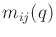denote an entry of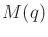. Suppose that the potential energy is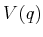. By performing the derivatives expressed in (13.136), the Euler-Lagrange equation can be expressed asscalar equations of the form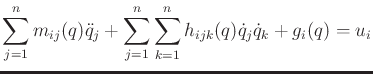(13.140)

in which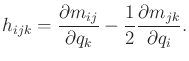(13.141)

There is one equation for eachfromto. The components of (13.140) have physical interpretations. The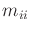coefficients represent the inertia with respect to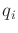. The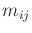represent the affect on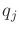of accelerating. The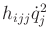terms represent the centrifugal effect induced onby the velocity of. The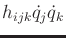terms represent the Coriolis effect induced onby the velocities ofand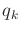. The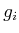term usually arises from gravity.

An alternative to (13.140) is often given in terms of matrices. It can be shown that the Euler-Lagrange equation reduces to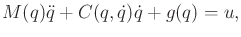(13.142)

which representsscalar equations. This introduces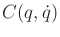, which is an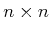Coriolis matrix. It turns out that many possible Coriolis matrices may produce equivalent different constraints. With respect to (13.140), the Coriolis matrix must be chosen so that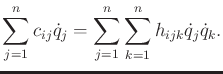(13.143)

Using (13.141),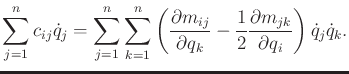(13.144)

A standard way to determineis by computing Christoffel symbols. By subtracting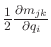from the inside of the nested sums in (13.144), the equation can be rewritten as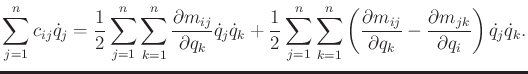(13.145)

This enables an element ofto be written as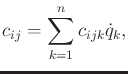(13.146)

in which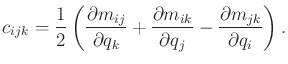(13.147)

This is called a Christoffel symbol, and it is obtained from (13.145). Note that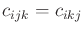. Christoffel symbols arise in the study of affine connections in differential geometry and are usually denoted as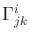. Affine connections provide a way to express acceleration without coordinates, in the same way that the tangent space was expressed without coordinates in Section 8.3.2. For affine connections in differential geometry, see ; for their application to mechanics, see .

Subsections
Steven M LaValle 2020-08-14